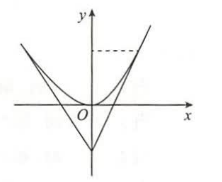(1) 求由这两条直线与抛物线 $y=x^2$ 所围成的平面图形绕 $y$ 轴旋转一周所得到的旋转体的表面积:
（2）求上述旋转体的体积
【答案】 【解】先求切线方程: 设切点为 $\left(x_0, x_0^2\right)$, 则切线方程为 $y-x_0^2=2 x_0\left(x-x_0\right)$, 因切线过 $(0,-1)$ 点, 故 $-1-x_0^2=-2 x_0^2$, 解得 $x_0=\pm 1$, 于是两条切线方程分别为 $y=2 x-1, y=-2 x-1$.
(I) 由于所围图形关于 $y$ 轴对称, 于是只需求 $y$ 轴右侧的图形绕 $y$ 轴旋

\begin{aligned} S_1 &=2 \pi \int_{-1}^1 \frac{1}{2}(y+1) \sqrt{1+\left\{\left[\frac{1}{2}(y+1)\right]^{\prime}\right\}^2} \mathrm{~d} y \\ &=\frac{\sqrt{5}}{2} \pi \int_{-1}^1(y+1) \mathrm{d} y=\sqrt{5} \pi . \end{aligned}

$$S_2=2 \pi \int_0^1 \sqrt{y} \sqrt{1+\left[(\sqrt{y})^{\prime}\right]^2} \mathrm{~d} y=\pi \int_0^1 \sqrt{1+4 y} \mathrm{~d} y=\frac{\pi}{6}(5 \sqrt{5}-1) .$$

$$S=S_1+S_2==\sqrt{5} \pi+\frac{\pi}{6}(5 \sqrt{5}-1)=\frac{\pi}{6}(11 \sqrt{5}-1) .$$
(II) 由于所围图形关于 $y$ 轴对称, 所以旋转体的体积为
$$V=V_1-V_2=\pi \int_{-1}^1\left[\frac{1}{2}(y+1)\right]^2 \mathrm{~d} y-\pi \int_0^1(\sqrt{y})^2 \mathrm{~d} y=\frac{2}{3} \pi-\frac{1}{2} \pi=\frac{\pi}{6} .$$